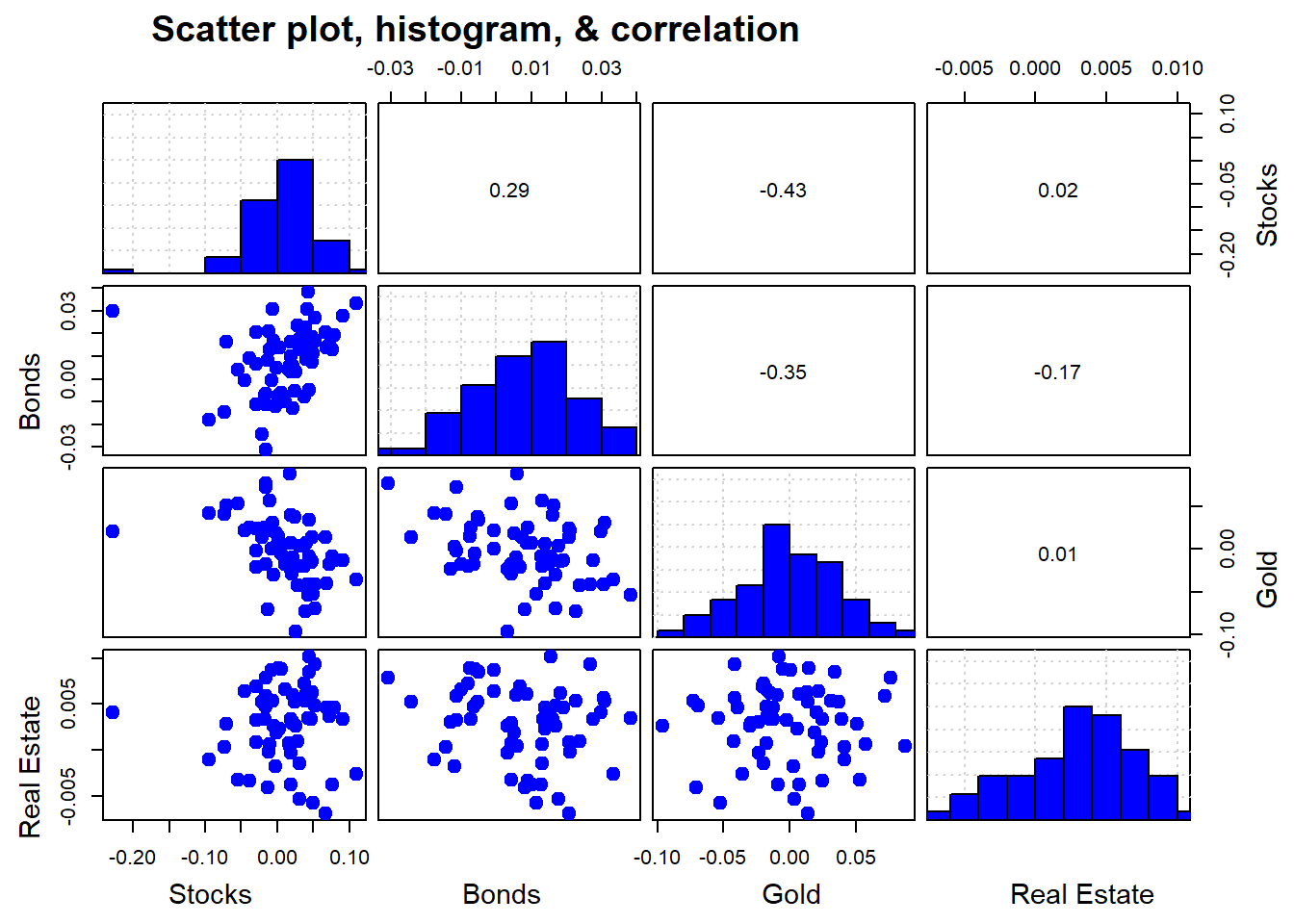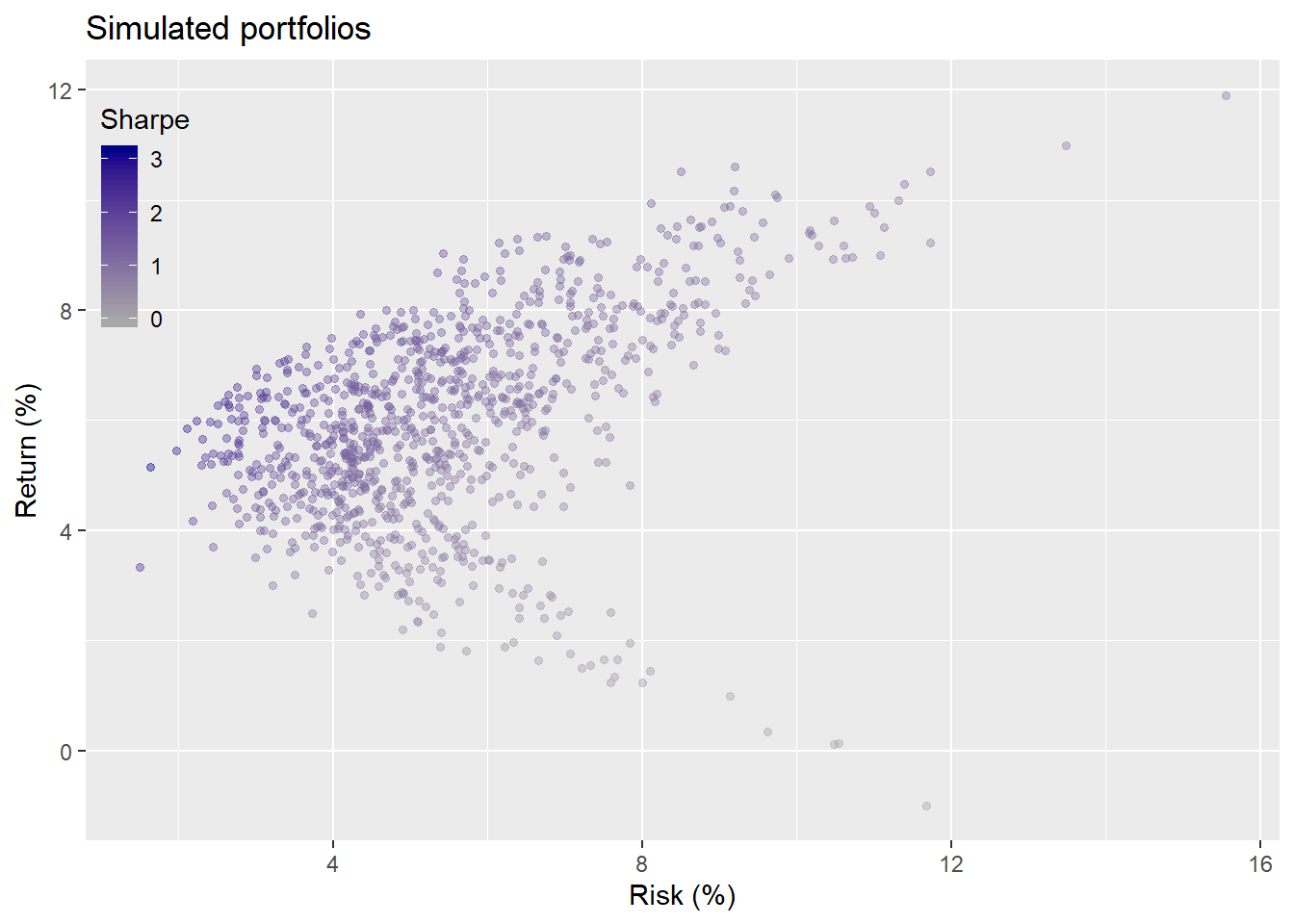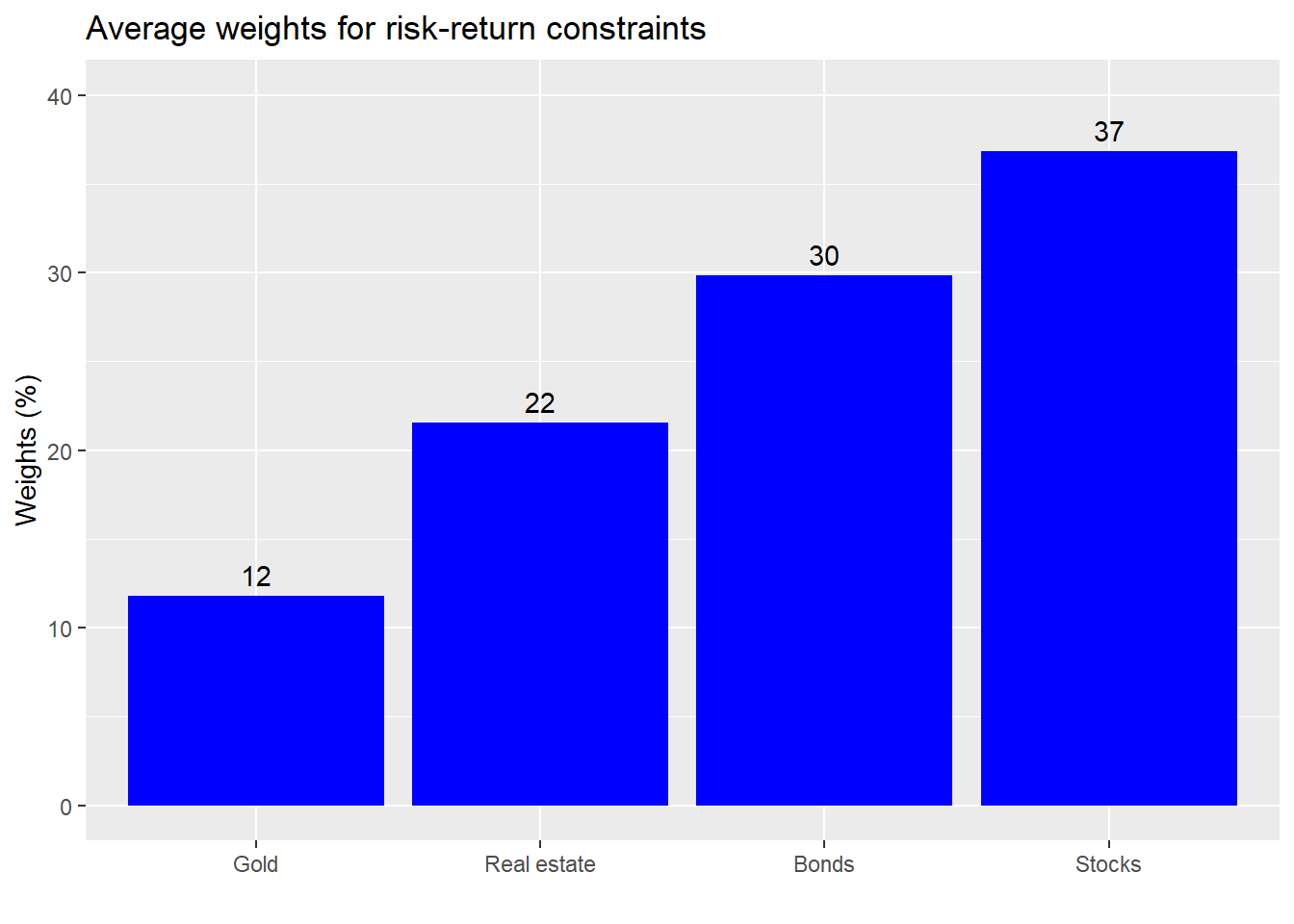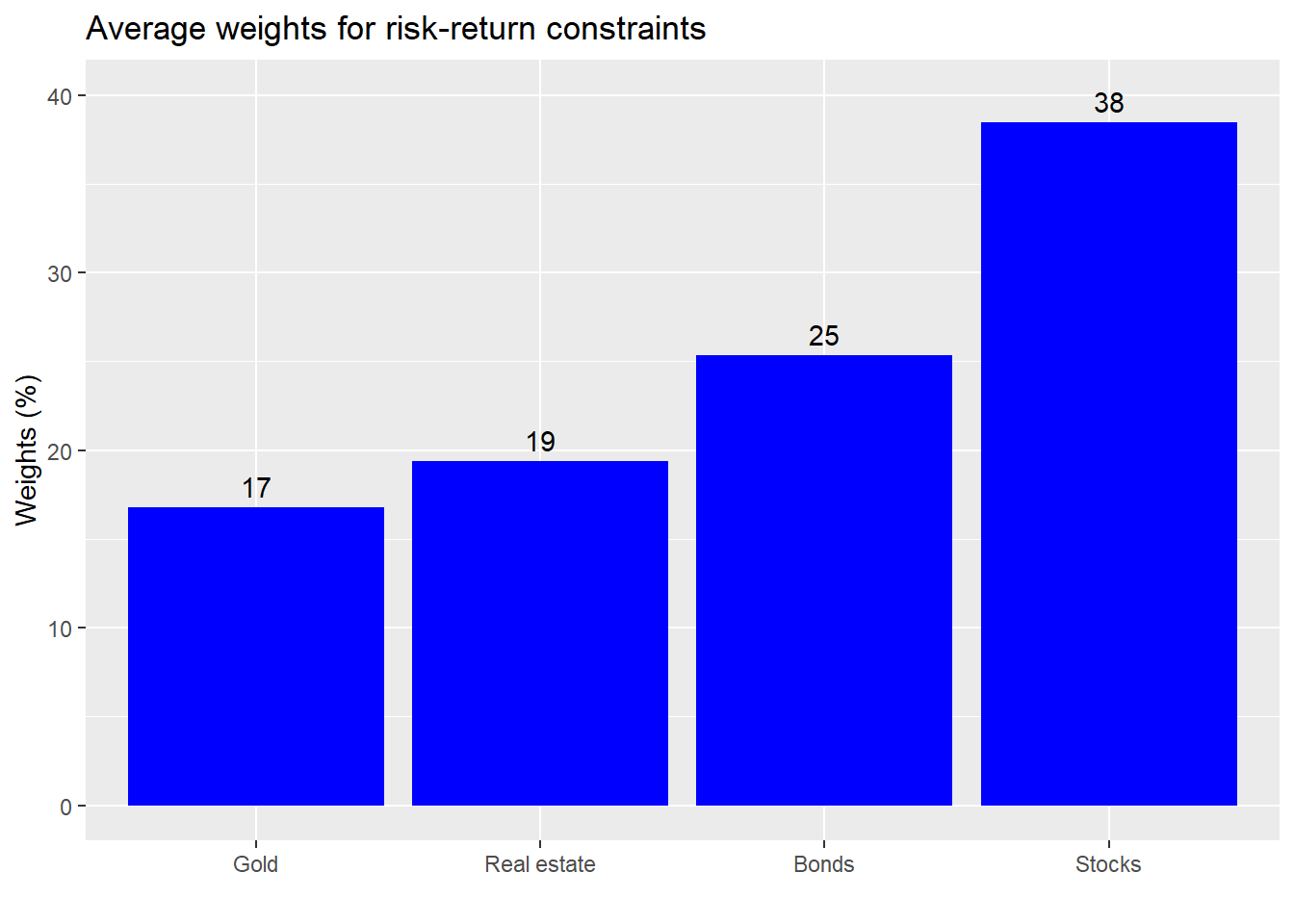Want to share your content on R-bloggers? click here if you have a blog, or here if you don't.

In our last post, we compared the three most common methods used to set return expectations prior to building a portfolio. Of the three—historical averages, discounted cash flow models, and risk premia models—no single method dominated the others on average annual returns over one, three, and five-year periods. Accuracy improved as the time frame increased. Additionally, aggregating all three methods either by averaging predictions, or creating a multivariate regression from the individual explanatory variables, performed better than two out of the three individual methods. Nonetheless, on a five-year time horizon, the historical average method enjoyed the highest accuracy even against an ensemble of all methods.

In this post, we’ll take the historical average method and apply it to building an portfolio and then test that portfolio against actual results. Our study will proceed as follows:

• Gather a representative group of assets.
• Calculate the prior five-year returns as the method to set expectations.
• Simulate allocation weights
• Choose an appropriate weighting scheme for the prospective portfolio
• Deploy the portfolio over the next five years without rebalancing
• Compare to actual results

Before we begin, we want to highlight that we’re now including a python version of the code that replicates the analysis and graphs we achieve with R. You’ll find it after the R code at the bottom of the post.

#### Asset gathering

Choosing a representative group of assets is not a trivial matter for reasons we’ve discussed in previous posts, not least of which is finding publicly available series that have a sufficiently long record. In the past, we’ve used large, liquid ETFs. Unfortunately, start dates vary, which means the amount of data is limited by the ETF with the shortest trading record. On the flip side, getting long data series in some cases implies using data that didn’t exist at the time. Those 100-year analyses of the S&P 500 are fine for academic purposes. But don’t reflect investing reality since the index didn’t exist before the 1950s and wasn’t broadly investable by individuals until the great John Bogle created the first index fund in the mid-1970s. And no one wanted to buy it at first anyway!

We also want total return indices, as these reflect the benefits of dividends and interest that a real owner would receive. On this account, we scoured the St. Louis Fed’s database (FRED) to come up with four representative asset classes: stocks, bonds, commodities, and real estate as well as the risk-free rate. These are the Wilshire 5000 total market stock index, the ICE Bank of America investment grade corporate bond index, the gold index, the Case-Shiller Home price index, and the five-year Treasury note constant maturity index. The earliest start date for which all values are available is 1987, so this is a pretty good length. The downside is that apart from gold and Treasuries, these indices weren’t investable for much of the period (Wilshire 5000) or at all (Case-Shiller). So we’re sacrificing reality for illustration.

Whateeer the case, let’s pull that data and then start with exploratory data analysis. We show the scatter plot, histograms, and correlation of returns in the chart below.1Figure 1: Source: St. Louis Fed

We see that the correlations between the various asset classes is relatively low; returns distributions are dissimilar; and the scatter plots show limited linear relationships.

#### Calculate returns and simulate portfolios

Now we’ll run a 1,000 simulations in which we randomly choose weights for the different asset classes and graph the resulting return (y-axis) against risk (x-axis) associated with those portfolios. We modulate the hue of each portfolio by its return-to-risk, or Sharpe, ratio—darker is higher.The graph seems fairly well distributed with a roughly balanced shape. The question now is what allocation based on this simulation is likely to produce our required return and risk tolerance? Hard to answer without a mandate or return target such as a benchmark or sustainable spending goal. Instead, we could put ourselves in the shoes of someone in 1992 (the start of the first portfolio) and imagine what she or he would be satisfied to achieve. The average annualized return for stocks from 1970-1992 was around 10% with a standard deviation close to 16%, yielding a Sharpe ratio of 0.125 to 0.6 depending on whether or not you adjust for Treasury yields (the risk-free rate) and which duration you use.

#### Choose weighting scheme

Assuming we want equity-like returns with lower risk (what’s the point of diversification anyway!), we might search for returns that are above the 7% range with risk below 10%. We see from the graph above that this doesn’t cover a lot of the portfolios, so such constraints might not be realistic, but let’s see what the average weighting would be to achieve such a result.The weights are rather balanced and not outlandish. While one could quibble with a weighting here or there, it would be hard to argue that this a “bad” or inherently risky allocation. Instead, one might argue that the allocation to stocks isn’t high enough.

#### Deploy portfolio and compare

Now we’ll see what our portfolio would like vs. what actually happened over the next five years. In this case, we’ll assume we buy all of the assets at the prescribed weights at the beginning of the period and hold them until the end without any rebalancing. Clearly a highly artificial assumption, but we need to start somewhere. We’ll indicate our portfolio by the red dot in the scatter.A very good result. Our portfolio looks close to the top end of the range for it’s risk. Portfolio returns averaged about 9% annually with risk of about 4%, which is actually quite unusual. (Then again this was the internet boom.) Adjusting for the risk-free rate based on constant maturity five-year Treasury yields, the Sharpe ratio for the portfolio is 0.61, which is quite good for such a naive allocation.

Seeing that the portfolio performed so well, should we keep our current allocation or adjust it? And if we adjust it, should we base the simulations on the most recent five-years of data or the full period? The answer: let R do the heavy lifting while we survey the results.

If we keep the same constraints on returns of not less than 7% and risk not greater than 10%, the average weighting based on the previous five-years of data yields the following.The weights appear to be relatively the same in terms of allocations to stocks and bonds, but gold gets a much higher allocation on the back of real estate. We’ll save space by omitting the simulation based on 10-year returns; it’s not much different than the five-year. Nonetheless, it might be instructive to graph the simulation on 10-year returns and compare it to the portfolio’s results, the red dot.What the fudge? The portfolio achieved results that are out of the bounds of the simulation? This is not as crazy as it looks and is actually quite informative. Recall, the portfolio’s results are only for the second five-year period. Hence, if returns are back-end loaded, as appeared to be the case, then that subset could appear to be outside the realm of possibility since the earlier returns weigh down the averages for the entire period.

Second, what this suggests is that our weighting scheme may have just been lucky due to the timing of our allocation. Recall, we bought the entire portfolio on day one. We’d need to test whether a more measured allocation framework would have yielded different results. But that will have to wait for another post. Critically, the levitating red dot begs the question whether we want alter our allocations or constraints. Maybe returns of not less than 7% and risk of not more than 10% are unrealistic. Say we lowered it to a return not less than 6% and a risk not more than 10%. What would the average allocations approximate? We graph the results based on the last ten years of data.This allocation isn’t much different from the one above. The weighting to stocks declines in favor of gold. Real estate returns to its original allocation. This is instructive because it shows us how an aggregate weighting scheme may not produce logical results. Few investors would consider putting more than 5% of their portfolio in a commodity like gold unless they had a strong view on inflation or potential econmic shocks. Importantly, what’s causing the shift to gold? Is it risk or return? As a perceptive reader explained to us previously, returns generally have a greater impact on allocation than volatility. And this case proves that out, as gold exhibited a negative return on average, while its volatility was the second highest of the group.

A more reasonable allocation would be to increase one’s exposure to bonds and to keep the gold allocation below 10%. We’ll save the analysis of ranges of allocations for a later post. If this were reality, it might make more sense to keep the original allocation, but to be prepared for lower returns going forward. Yet, this is about illustration. So let’s look at two allocation strategies. Keeping the prior one (why mess with a good thing?) and the one based on longer term data.Interestingly, the different allocations didn’t result in meaningfully different results when looking at the graph. We see that in general, returns averaged about 5%, below our threshold constraint. This was mainly due to the bursting of the tech bubble in 2000 and the 2001 recession. Still our portfolio performed better than 80% of the simulations. Better to be lucky than smart.

#### Discussion

We could keep running simulations to the end the period. But we’ll end it here. The code below provides functions that should allow one to produce simulations up to the present relatively straightforward.

What are the key takeaways? While it’s tough to generalize on such a small sample, it’s safe to say that portfolio results are decidedly at the mercy of what actually occurs. Those strong risk-adjusted returns in the first test period were likely due to the bull market rather than our allocation. Additionally, the gimlet-eyed reader will notice how the shape of the portfolio simulations changed with the different periods. In the first simulation, one could almost trace a sideways parabola along the edges of the dots. The last looked more like a lop-sided V. Examining these results gives us a good launchpad for further analysis. What’s next then? In future posts, we’ll

• Run performance analysis including attribution and comparisons to equal weighting
• Compare simulated allocations to optimized ones.
• Examine different rebalancing schemes
• Analyze how allocation tranching (not buying all at once) affects performance.
• Investigate how to allocate a portfolio whose estimates are prone to sampling error.

If you’d prefer reading about one of these analyses sooner, rather than later, drop us an email at the address below. Until next time, the R followed by the python code for all the analyses and graphs are below.

```## Coded in R 3.6.2

suppressPackageStartupMessages({
library(tidyquant)
library(tidyverse)
})

# Create symbol vectors
symbols <- c("WILL5000INDFC", "BAMLCC0A0CMTRIV", "GOLDPMGBD228NLBM", "CSUSHPINSA", "DGS5")
sym_names <- c("stock", "bond", "gold", "realt", "rfr")

# Get symbols
getSymbols(symbols, src="FRED", from = "1970-01-01", to = "2019-12-31")

# Merge xts objects and resample to monthly
index <- merge(WILL5000INDFC, BAMLCC0A0CMTRIV, GOLDPMGBD228NLBM, CSUSHPINSA, DGS5)
index <- na.locf(index)
colnames(index) <- sym_names

idx_mon <- to.monthly(index, indexAt = "lastof", OHLC=FALSE)
idx_mon <- idx_mon["1987/2019"]

# Create data frame
df <- data.frame(date = index(idx_mon), coredata(idx_mon)) %>%
mutate_at(vars(-c(date, rfr)), function(x) x/lag(x)-1) %>%
mutate(rfr = rfr/100)

## Plot data
# Note special thanks to Stackoverflow and user eipi10 for the code to make a ggpairs-like plot without suffering constraints of that package. Simple manual color changes are not workable!
# https://stackoverflow.com/questions/13367248/pairs-move-labels-to-sides-of-scatter-plot

panel.hist <- function(x, ...)
{
usr <- par("usr"); on.exit(par(usr))
par(usr = c(usr[1:2], 0, 1.5) )
h <- hist(x, plot = FALSE)
breaks <- h\$breaks; nB <- length(breaks)
y <- h\$counts; y <- y/max(y)
rect(breaks[-nB], 0, breaks[-1], y, panel.first = grid(),...)
}

panel.pearson <- function(x, y, ...) {
horizontal <- (par("usr") + par("usr")) / 2;
vertical <- (par("usr") + par("usr")) / 2;
text(horizontal, vertical, round(cor(x,y)+0.009, 2))}

pairs(df[2:61 , 2:5],
col = "blue",
pch = 19,
cex = 1.5,
labels = NULL,
gap = 0.5,
diag.panel = panel.hist,
upper.panel = panel.pearson)

title("Scatter plot, histogram, & correlation", adj = 0, line = 3)

x.coords = par('usr')[1:2]
y.coords = par('usr')[3:4]

# Offset is estimated distance between edge of plot area and beginning of actual plot
x.offset = 0.03 * (x.coords - x.coords)
xrng =  (x.coords - x.coords) - 2*x.offset
x.width = xrng/4

y.offset = 0.028 * (y.coords - y.coords)
yrng =  (y.coords - y.coords) - 2*y.offset
y.width = yrng/4

# x-axis labels
text(seq(x.coords + x.offset + 0.5*x.width, x.coords - x.offset - 0.5*x.width,
length.out=4), 0,
c("Stocks","Bonds","Gold","Real Estate"),

# y-axis labels
text(x.coords, seq(y.coords + y.offset + 0.5*y.width, y.coords - 3*y.offset - 0.5*y.width,
length.out=4),
rev(c("Stocks","Bonds","Gold","Real Estate")),
srt=90,  # rotates text to be parallel to axis
cex=.9)

## Portfolio simulation

# Weighting that ensures more variation and random weighthing to stocks
set.seed(123)

# Function for simulation and graph
port_sim <- function(df, sims, cols){

if(ncol(df) != cols){
print("Columns don't match")
break
}

# Create weight matrix
wts <- matrix(nrow = sims, ncol = cols)

for(i in 1:sims){
a <- runif(cols,0,1)
b <- a/sum(a)
wts[i,] <- b
}

# Find returns
mean_ret <- colMeans(df)

# Calculate covariance matrix
cov_mat <- cov(df)

# Calculate random portfolios
port <- matrix(nrow = sims, ncol = 2)
for(i in 1:sims){
port[i,1] <- as.numeric(sum(wts[i,] * mean_ret))
port[i,2] <- as.numeric(sqrt(t(wts[i,] %*% cov_mat %*% wts[i,])))
}

colnames(port) <- c("returns", "risk")
port <- as.data.frame(port)
port\$Sharpe <- port\$returns/port\$risk*sqrt(12)

max_sharpe <- port[which.max(port\$Sharpe),]

graph <- port %>%
ggplot(aes(risk*sqrt(12)*100, returns*1200, color = Sharpe)) +
geom_point(size = 1.2, alpha = 0.4) +
scale_color_gradient(low = "darkgrey", high = "darkblue")+
labs(x = "Risk (%)",
y = "Return (%)",
title = "Simulated portfolios")

out <- list(port = port, graph = graph, max_sharpe = max_sharpe, wts = wts)

}

## Run simulation and plot
port_sim_1 <- port_sim(df[2:61,2:5],1000,4)

port_sim_1\$graph +
theme(legend.position = c(0.05,0.8), legend.key.size = unit(.5, "cm"),
legend.background = element_rect(fill = NA))

# Create function to calculate portfolio weights based on constraints and a graph
port_select_func <- function(port, return_min, risk_max, port_names){
port_select  <-  cbind(port\$port, port\$wts)
risk_sd <- sd(port_sim_1\$port\$risk)

port_wts <- port_select %>%
mutate(returns = returns*12,
risk = risk*sqrt(12)) %>%
filter(returns >= return_min,
risk <= risk_max) %>%
summarise_at(vars(4:7), mean) %>%
`colnames<-`(port_names)

graph <- port_wts %>%
rename("Stocks" = 1,
"Bonds" = 2,
"Gold" = 3,
"Real esate" = 4) %>%
gather(key,value) %>%
ggplot(aes(reorder(key,value), value*100 )) +
geom_bar(stat='identity', position = "dodge", fill = "blue") +
geom_text(aes(label=round(value,2)*100), vjust = -0.5) +
scale_y_continuous(limits = c(0,40)) +
labs(x="",
y = "Weights (%)",
title = "Average weights for risk-return constraints")

out <- list(port_wts = port_wts, graph = graph)

out

}

## Run selection function
results_1 <- port_select_func(port_sim_1,0.07, 0.1, sym_names[1:4])
results_1\$graph

## Instantiate weighting
fut_wt <- results_1\$port_wts

## Create rebalancing function
rebal_func <- function(act_ret, weights){
tot_ret <- 1
ret_vec <- c()
for(i in 1:60){
wt_ret <- act_ret[i,]*weights # wt'd return
ret <- sum(wt_ret) # total return
tot_ret <- tot_ret * (1+ret) # cumulative return
ret_vec[i] <- ret
weights <- (weights + wt_ret)/(sum(weights)+ret) # new weight based on change in asset value
}
ret_vec
}

## Run function and create actual portfolio
ret_vec <- rebal_func(df[61:121,2:5], fut_wt)

port_act <- data.frame(returns = mean(ret_vec),
risk = sd(ret_vec),
sharpe = mean(ret_vec)/sd(ret_vec)*sqrt(12))

# Run simulation on recent five-years
port_sim_2 <- port_sim(df[62:121,2:5], 1000, 4)

# Graph simulation with actual portfolio return
port_sim_2\$graph +
# geom_abline(slope = test1\$max_sharpe\$Sharpe, color = "blue", linetype = "dashed") +
geom_point(data = port_act,
aes(risk*sqrt(12)*100, returns*1200), size = 4, color="red") +
theme(legend.position = c(0.05,0.8), legend.key.size = unit(.5, "cm"),
legend.background = element_rect(fill = NA))

# Run function on next five years implied weight
results_2 <- port_select_func(port_sim_2, 0.07, 0.1,sym_names[1:4])
results_2\$graph

# Run simulation on last 10 years
port_sim_2l <- port_sim(df[2:121,2:5], 1000,4)

# Graph simulation with actual results of last five years
port_sim_2l\$graph +
geom_point(data = port_act,
aes(risk*sqrt(12)*100, returns*1200), size = 4, color="red") +
theme(legend.position = c(0.05,0.8), legend.key.size = unit(.5, "cm"),
legend.background = element_rect(fill = NA))

## Run portfolio selection function on more conservative constraints and graph
resuls_2l_cons <- port_select_func(port_sim_2l, 0.06, 0.12, sym_names[1:4])
resuls_2l_cons\$graph

## Run two separate allocations on next five-years
results_2l <- port_select_func(port_sim_2l, 0.07, 0.1, sym_names[1:4])
ret_old_wt <- rebal_func(df[122:181, 2:5], fut_wt)
ret_new_wt <- rebal_func(df[122:181, 2:5], results_2l\$port_wts)

port_act_1_old <- data.frame(returns = mean(ret_old_wt),
risk = sd(ret_old_wt),
sharpe = mean(ret_old_wt)/sd(ret_old_wt)*sqrt(12))

port_act_1_new <- data.frame(returns = mean(ret_new_wt),
risk = sd(ret_new_wt),
sharpe = mean(ret_new_wt)/sd(ret_new_wt)*sqrt(12))

port_sim_3 <- port_sim(df[122:181,2:5], 1000, 4)

port_sim_3\$graph +
geom_point(data = port_act_1_old,
aes(risk*sqrt(12)*100, returns*1200), size = 4, color="red") +
geom_point(data = port_act_1_new,
aes(risk*sqrt(12)*100, returns*1200), size = 4, color="purple") +
theme(legend.position = c(0.05,0.8), legend.key.size = unit(.5, "cm"),
legend.background = element_rect(fill = NA))```

And for the pythonistas:

```# Coded in Python 3.7.4

import pandas as pd
import numpy as np
import matplotlib.pyplot as plt
%matplotlib inline

import seaborn as sns
plt.style.use('ggplot')
sns.set()

start_date = '1970-01-01'
end_date = '2019-12-31'
symbols = ["WILL5000INDFC", "BAMLCC0A0CMTRIV", "GOLDPMGBD228NLBM", "CSUSHPINSA", "DGS5"]
sym_names = ["stock", "bond", "gold", "realt", 'rfr']
filename = 'port_const.pkl'

try:
except FileNotFoundError:
data = web.DataReader(symbols, 'fred', start_date, end_date)
data.columns = sym_names

data_mon = data.resample('M').last()
df = data_mon.pct_change()['1987':'2019']
df.to_pickle(filename)

# Exploratory data analysis
sns.pairplot(df.iloc[1:61,0:4])
plt.show()

# Create function
class Port_sim:
def calc_sim(df, sims, cols):
wts = np.zeros((sims, cols))

for i in range(sims):
a = np.random.uniform(0,1,cols)
b = a/np.sum(a)
wts[i,] = b

mean_ret = df.mean()
port_cov = df.cov()

port = np.zeros((sims, 2))
for i in range(sims):
port[i,0] =  np.sum(wts[i,]*mean_ret)
port[i,1] = np.sqrt(np.dot(np.dot(wts[i,].T,port_cov), wts[i,]))

sharpe = port[:,0]/port[:,1]*np.sqrt(12)
best_port = port[np.where(sharpe == max(sharpe))]
max_sharpe = max(sharpe)

return port, wts, best_port, sharpe, max_sharpe

def graph_sim(port,sharpe):
plt.figure(figsize=(14,6))
plt.scatter(port[:,1]*np.sqrt(12)*100, port[:,0]*1200, marker='.',c=sharpe, cmap='Blues')
plt.colorbar(label='Sharpe ratio', orientation = 'vertical', shrink = 0.25)
plt.title('Simulated porfolios', fontsize=20)
plt.xlabel('Risk (%)')
plt.ylabel('Return (%)')
plt.show()

# Create simulation
np.random.seed(123)
port, wts, _, sharpe, _ = Port_sim.calc_sim(df.iloc[1:60,0:4],1000,4)

# Graph simulation
Port_sim.graph_sim(port, sharpe)

#### Portfolio constraint function
def port_select_func(port, wts, return_min, risk_max):
port_select = pd.DataFrame(np.concatenate((port, wts), axis=1))
port_select.columns = ['returns', 'risk', 1, 2, 3, 4]

port_wts = port_select[(port_select['returns']*12 >= return_min) & (port_select['risk']*np.sqrt(12) <= risk_max)]
port_wts = port_wts.iloc[:,2:6]
#     port_wts.columns = ["Stocks", "Bonds", "Gold", "Real estate"]
port_wts = port_wts.mean(axis=0)

def graph():
plt.figure(figsize=(12,6))
key_names = {1:"Stocks", 2:"Bonds", 3:"Gold", 4:"Real estate"}
lab_names = []
graf_wts = port_wts.sort_values()*100

for i in range(len(graf_wts)):
name = key_names[graf_wts.index[i]]
lab_names.append(name)

plt.bar(lab_names, graf_wts)
plt.ylabel("Weight (%)")
plt.title("Average weights for risk-return constraint", fontsize=15)

for i in range(len(graf_wts)):
plt.annotate(str(round(graf_wts.values[i])), xy=(lab_names[i], graf_wts.values[i]+0.5))

plt.show()

return port_wts, graph()

## Run function
results_wts, results_graph = port_select_func(port, wts, 0.05, 0.14)

## Create rebalancing function
def rebal_func(act_ret, weights):
tot_ret = 1
ret_vec = np.zeros(60)
for i in range(60):
wt_ret = act_ret.iloc[i,:].values*weights
ret = np.sum(wt_ret)
tot_ret = tot_ret * (1+ret)
ret_vec[i] = ret
weights = (weights + wt_ret)/(np.sum(weights) + ret)

return ret_vec

# Run rebalancing function and dictionary
ret_vec = rebal_func(df.iloc[61:121,0:4], results_wts)
port_act = {'returns': np.mean(ret_vec),
'risk': np.std(ret_vec),
'sharpe': np.mean(ret_vec)/np.std(ret_vec)*np.sqrt(12)}

#### Run simulation on next group
# Run simulation on recent five-years
np.random.seed(123)
port_2, wts_2, _, sharpe_2, _ = Port_sim.calc_sim(df.iloc[61:121,0:4],1000,4)

# Graph simulation with actual portfolio return
plt.figure(figsize=(14,6))
plt.scatter(port_2[:,1]*np.sqrt(12)*100, port_2[:,0]*1200, marker='.', c=sharpe, cmap='Blues')
plt.colorbar(label='Sharpe ratio', orientation = 'vertical', shrink = 0.25)
plt.scatter(port_act['risk']*np.sqrt(12)*100, port_act['returns']*1200, c='blue', s=50)
plt.title('Simulated portfolios', fontsize=20)
plt.xlabel('Risk (%)')
plt.ylabel('Return (%)')
plt.show()

#### Run selection function n next five years implied weight
results_wts_2, results_graph_2 = port_select_func(port_2, wts_2, 0.07, 0.1)

# Run simulation on recent five-years
np.random.seed(123)
port_2l, wts_2l, _, _, _ = Port_sim.calc_sim(df.iloc[1:121,0:4],1000,4)

# Graph simulation with actual portfolio return
plt.figure(figsize=(14,6))
plt.scatter(port_2l[:,1]*np.sqrt(12)*100, port_2l[:,0]*1200, marker='.', c=sharpe, cmap='Blues')
plt.colorbar(label='Sharpe ratio', orientation = 'vertical', shrink = 0.25)
plt.scatter(port_act['risk']*np.sqrt(12)*100, port_act['returns']*1200, c='red', s=50)
plt.title('Simulated portfolios', fontsize=20)
plt.xlabel('Risk (%)')
plt.ylabel('Return (%)')
plt.show()

# Run function on next five years implied weight
results_wts_2l, _ = port_select_func(port_2l, wts_2l, 0.06, 0.12)

# Run simulation on next five years with two different weightings
np.random.seed(123)
ret_old_wt = rebal_func(df.iloc[121:181, 0:4], fut_wt)
ret_new_wt = rebal_func(df.iloc[121:181, 0:4], results_wts_2l)

port_act_1_old = {'returns' : np.mean(ret_old_wt),
'risk' : np.std(ret_old_wt),
'sharpe' : np.mean(ret_old_wt)/np.std(ret_old_wt)*np.sqrt(12)}

port_act_1_new = {'returns' : np.mean(ret_new_wt),
'risk' : np.std(ret_new_wt),
'sharpe' : np.mean(ret_new_wt)/np.std(ret_new_wt)*np.sqrt(12)}

port_3, wts_3, _, _, _ = Port_sim.calc_sim(df.iloc[121:181, 0:4], 1000, 4)

plt.figure(figsize=(14,6))
plt.scatter(port_3[:,1]*np.sqrt(12)*100, port_3[:,0]*1200, marker='.', c=sharpe, cmap='Blues')
plt.colorbar(label='Sharpe ratio', orientation = 'vertical', shrink = 0.5)
plt.scatter(port_act_1_old['risk']*np.sqrt(12)*100, port_act_1_old['returns']*1200, c='red', s=50)
plt.scatter(port_act_1_new['risk']*np.sqrt(12)*100, port_act_1_new['returns']*1200, c='purple', s=50)
plt.title('Simulated portfolios', fontsize=20)
plt.xlabel('Risk (%)')
plt.ylabel('Return (%)')
plt.show()```

1. Note this isn’t the normal ggplot style we typically use. We struggled to work with the GGally package to get the right color scheme. After much frustration and plenty of Stackoverflow queries, we went with a base R solution. In the code, we give full credit to the R guru who’s code saved the day.